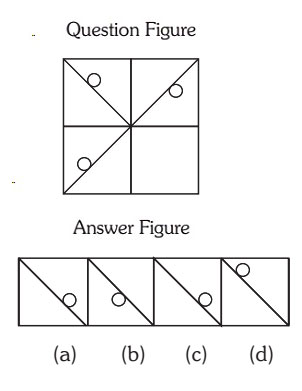#### Completion Of Incomplete Pattern

Direction: In each of the following questions, find out which of the answer figures (a), (b), (c) and (d) completes the figure?

1. Following Question has four alternatives, among which one completes the figures.1. Option (a) in Answer Figure.
2. Option (b) in Answer Figure.
3. Option (c) in Answer Figure.
4. Option (d) in Answer Figure.
##### Correct Option: B

Rod is moving 90 degree in clockwise direction. Move the Rod from 3rd box by 90 degree in clockwise direction.